Next: Binary Star Systems Up: Two-Body Dynamics Previous: Introduction

Reduced Mass

Suppose that our first object is of mass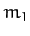, and is located at position vector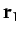. Likewise, our second object is of mass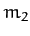, and is located at position vector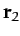. Let the first object exert a force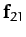on the second. By Newton's third law, the second object exerts an equal and opposite force,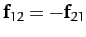, on the first. Suppose that there are no other forces in the problem. The equations of motion of our two objects are thus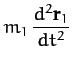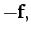(322)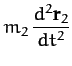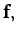(323)

where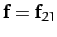.

Now, the center of mass of our system is located at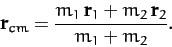(324)

Hence, we can write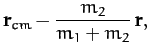(325)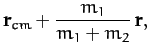(326)

where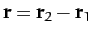. Substituting the above two equations into Equations (322) and (323), and making use of the fact that the center of mass of an isolated system does not accelerate (see Section 2.6), we find that both equations yield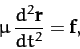(327)

where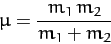(328)

is called the reduced mass. Hence, we have effectively converted our original two-body problem into an equivalent one-body problem. In the equivalent problem, the force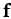is the same as that acting on both objects in the original problem (modulo a minus sign). However, the mass,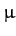, is different, and is less than either ofor(which is why it is called the reduced'' mass).Next: Binary Star Systems Up: Two-Body Dynamics Previous: Introduction
Richard Fitzpatrick 2011-03-31